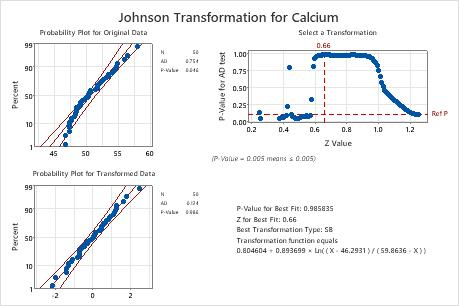# Interpret the key results for the Johnson Transformation

Complete the following steps to interpret the Johnson Transformation. Key output includes probability plots, p-values, and the transformation function.

## Step 1: View the fit of the normal distribution

Use the normal probability plots to assess how closely the original and transformed data follow the normal distribution.

If the Johnson transformation is effective and the normal distribution is a good fit for the transformed data, the points on the plot for the transformed data should closely follow the fitted normal distribution line. Departures from the straight line indicate that the fit is unacceptable and that the Johnson transformation is not effective.
In addition to the probability plot, use the AD p-values to evaluate the distribution fit. For more information, go to Interpret all statistics and graphs for Johnson Transformation and click P-Value.
###### Note

If the original data are normally distributed, Minitab displays only a single probability plot and does not perform the Johnson transformation.

## Step 2: Assess the fit of the normal distribution

Use the p-value to assess whether you can assume that the original and transformed data follow the normal distribution.

Compare the p-value to the alpha level. To evaluate the distribution fit, an alpha of 0.10 is often used.
• A p-value that is less than alpha indicates that the normal distribution is not a good fit.
• A p-value that is greater than or equal to alpha indicates that there is not enough evidence of a poor distribution fit. You can assume the data follow the normal distribution.

If the Johnson transformation is effective, the p-value for the transformed data is greater than alpha.

###### Important

Use caution when you interpret results from a very small or a very large sample. If you have a very small sample, a goodness-of-fit test may not have enough power to detect significant deviations from the distribution. If you have a very large sample, the test may be so powerful that it detects even small deviations from the distribution that have no practical significance. Use the probability plots in addition to the p-values to evaluate the distribution fit.Key Result: P-ValueIn these results, the p-value (0.046) for the original data is less than alpha (0.10), which indicates that the original calcium data are not normal. For the transformed data, the p-value (0.986) is greater than alpha. Therefore, you can assume that the transformed data follow a normal distribution.

## Step 3: Examine the transformation function

Minitab displays the parameters of the Johnson transformation function that produces the best fit. Minitab uses this function to transform the original data.

For example, suppose the Johnson transformation function is 0.762475 + 0.870902 × Ln((X – 46.3174 ) / (59.6770 – X)). If the original data value for X is 50, then the transformed data value of 50 is calculated as 0.762475 + 0.870902 × Ln((50 – 46.3174) / (59.6770 – 50)), which equals –0.07893.

###### Note

To store all the transformed data values in the worksheet, enter a storage column when you perform the analysis.

For more information on the algorithm that Minitab uses to define the Johnson transformation function, go to Methods and formulas for transformations in Individual Distribution Identification and click "Methods and formulas for the Johnson Transformation".